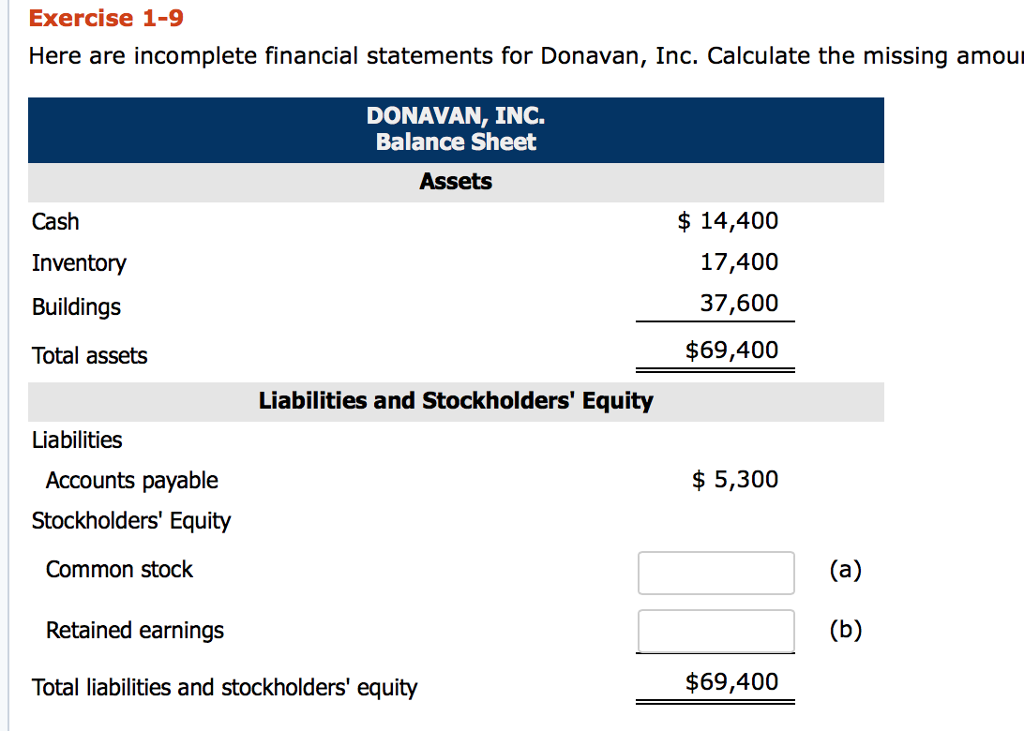# Here are incomplete financial statements for donavan. inc. calculate the missing amounts.

Here are incomplete financial
statements for Donavan, Inc. Calculate the missing amounts.Exercise 1-9 Here are incomplete financial statements for Donavan, Inc. Calculate the missing amour DONAVAN, INC. Balance Sheet Assets Cash Inventory Buildings Total assets \$ 14,400 17,400 37,600 \$69,400 Liabilities and Stockholders’ Equity Liabilities \$5,300 Accounts payable Stockholders’ Equity Common stock Retained earnings Total liabilities and stockholders’ equity \$69,400

e.
Net income: This is the difference of total revenues and total
expenses.
Beginning retained earnings + Net income – Dividends = Ending
retained earnings
19,400 + Net income – 4,160 = 34,400
Net income = 34,400 – 19,400 + 4,160

d.
Net income is calculated as above.
Net income = \$19,160 (Answer)
c.
Cost of goods sold is associated with all sorts of direct cost
for production.
Revenues – Cost of goods sold – Salaries = Net income
85,500 – Cost of goods sold – 10,410 = 19,160
Cost of goods sold = 85,500 – 10,410 – 19,160